# GRE Math Circles in Detail with Examples

Circle is a geometrical figure that always confuses all aspirants of the GRE Test, since many geometrical figures combined with circles makes it complex.

A circle is a collection of points that are present at equal distances from a common point which is known as the center of the circle. The equidistant length between the center and the collection of points is known as the radius (r).

And if a line is drawn connecting two points of circle, passing through center then it is known as diameter (d) of the circle. Alternatively, twice of radius is equal to the diameter.

Last comes the circumference of the circle and can be calculated as pi times diameter (d or pi times twice of radius (2r). The universal value defined for pi is 22/7 or approximately 3.14.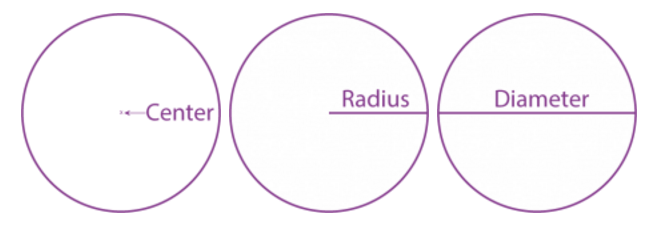Let us look at a few definitions associated with the circle.

Arc: It is a curved line which is a part of the circumference of the circle.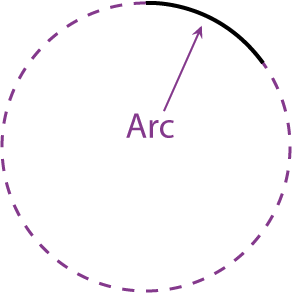Sector: It is like a slice of cake (a circle wedge)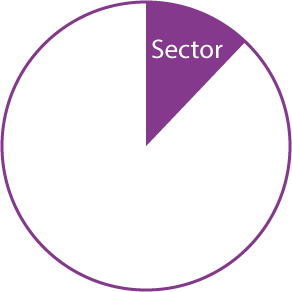Chord: It is a line segment inside the circle that touches 2 points on the circumference of the circle which divides the entire circle into two segments. The largest possible chord within a circle is the diameter.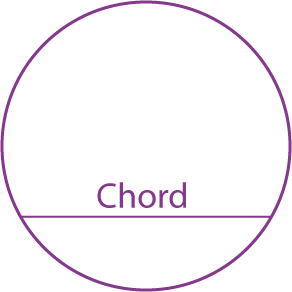Tangent of the circle: It is a line perpendicular to the radius which touches the circle at one and ONLY one point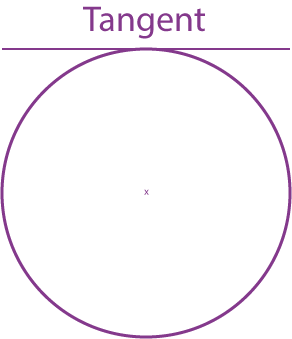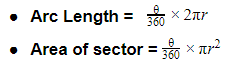where is the central angle for that arc length and is in degree.

Note: In GRE, angles will be given and tested only in degrees, not in radians.

The above two formulae can be combined and written in the form of part by the whole relationship as follows: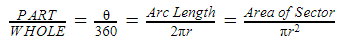Angle in a semi-circle made by the diameter is always 90°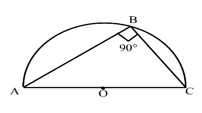The angles made by the same chord in the same segments are always equal to each other and a° + b° = 180°.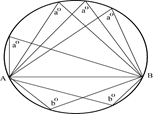The angle subtended from the chord at the center is always twice of the angle subtended from the same chord on the circumference.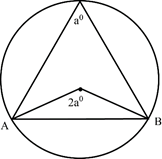Thus we hope these formulas assist to tackle this section of the GRE syllabus for quantitative reasoning.

Want to know more about GRE Quantitative section? Read our other GRE Quant Articles:

BYJU’S will be glad to help you in your GRE preparation journey. You can ask for any assistance related to GRE from us by just giving a missed call at 08884544444, or you can drop an SMS. You can write to us at gre@byjus.com.